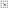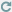## 期刊目錄列表 - 31～41期(1986-1996) - 第四十一期 (1996)Directory回上一頁

##### 一個解圓滑凸規劃的沿路徑內點法

Smooth convex programming, path-following interior point algorithm, complementarity problem, maximal complementary solution 本文主要在探討數學規劃中，近年來常被用來找近似解的內點法。在本論文中我們推廣Monteiro和Adler的沿路徑內點法 (path-following interior point algorithm) 以求解圓滑凸規劃問題，並分析探討其運算次數 (arithmetic operation) 之複雜性 (complexity)，在原問題有一嚴格可行解的條件下，我們證明這種內點法僅需要O( ) 迭代次數 (iterations)，且整個運算過程僅需O(n3﹐l) 個算數運算(arithmetic operations)。 其結果應用在凸二次規劃 (convex quadratic programming) 或 線性規劃 (linear programming) 問題時是最理想化的。更進一步地，我們的 內點法所產生的每一極限點都是其對應的互補問題 (complementarity problem) 的最大互補解。

## Journal directory listing - Volume 31-41 (1986-1996) - Volume 41 (1996)Directory Top

##### A Path-Following Interior Point Algorithm for Smooth Convex Programming
Author: Liang-Ju Chu(Department of Mathematics, National Taiwan Normal University)

Abstract：

We extend the Monteiro-Adler path-following interior point algorithm for solving smooth convex programming. Under a kind of strict feasibility assumption, we show that the algorithm under modification requires a total ofnumber of iterations, and the total arithmetic operations are not more than, where t is the initial input size. As an application to usual linear or convex quadratic programming, this algorithm solves the pair of primal and dual problems in at mostiterations, and the total arithmetic operations are shown to be of the order of, where L is the input size. Moreover, we show that any sequencegenerated by the algorithm is bounded, and that every cluster point is a maximal complementary solution in the sense of McLinden [16,17].

Keywords：Smooth convex programming, path-following interior point algorithm, complementarity problem, maximal complementary solution